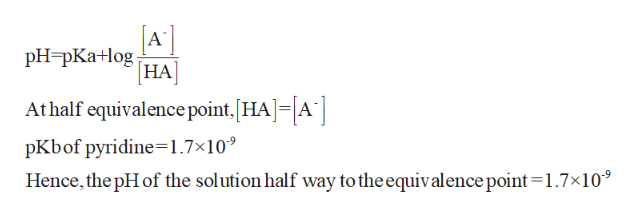# A 25.0-mL sample of 0.125 M pyridine is titrated with 0.100 MHCl. a.) Calculate the pH at 10 mL of added acid. b.)Calculate the pH at 20 mL of added acid. c.) Calculate the pH at equivalence point.d.) Calculate the pH at one-half equivalence point.

Question
391 views

A 25.0-mL sample of 0.125 M pyridine is titrated with 0.100 MHCl.

a.) Calculate the pH at 10 mL of added acid.

b.)Calculate the pH at 20 mL of added acid.

c.) Calculate the pH at equivalence point.

d.) Calculate the pH at one-half equivalence point.

check_circle

Step 1

C)

At halfway to the equivalent point,help_outlineImage TranscriptioncloseA PH PКа+og [НА] At half equivalence point, HAA pKbof pyridine-1.7x10 Hence, the pHof the solution half way to the equivalence point 1.7x10 fullscreen
Step 2

### Want to see the full answer?

See Solution

#### Want to see this answer and more?

Solutions are written by subject experts who are available 24/7. Questions are typically answered within 1 hour.*

See Solution
*Response times may vary by subject and question.
Tagged in

### Chemistry